# Multiplying Fractions Worksheet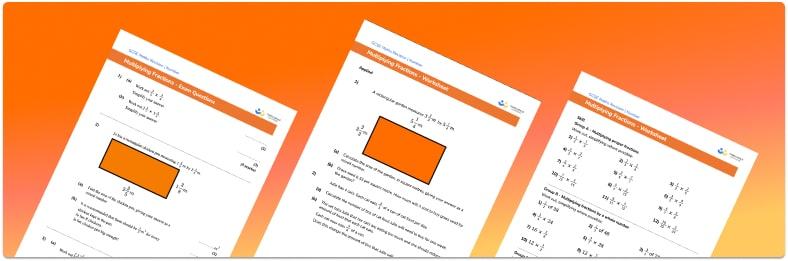• Section 1 of the multiplying fractions problems worksheet contains 20+ skills-based multiplying fractions questions, in 3 groups to support differentiation
• Section 2 contains 4 applied multiplying fractions questions with a mix of multiplying fractions word problems and deeper problem solving questions
• Section 3 contains 4 foundation and higher level GCSE exam style multiplying fractions questions
• Answer keys and a mark scheme for all multiplying fractions questions are provided
• Questions follow variation theory with plenty of opportunities for students to work independently at their own level
• All questions created by fully qualified expert secondary maths teachers
• Suitable for GCSE maths revision for AQA, OCR and Edexcel exam boards

• This field is for validation purposes and should be left unchanged.

You can unsubscribe at any time (each email we send will contain an easy way to unsubscribe). To find out more about how we use your data, see our privacy policy.

### Multiplying fractions a glance

Multiplication of fractions is more straightforward than adding or subtracting fractions, because a common denominator is not required if the question includes different denominators. It is important however that students are confident with equivalent fractions and simplifying fractions.

To multiply two fractions, simply multiply the numerators and multiply the denominators. The answer should always be written in its simplest form by cancelling common factors from the numerator and denominator.

A mixed number (or mixed fraction) has both an integer (whole number) part and a proper fraction part. To multiply mixed numbers we need to convert the mixed number into an improper fraction in its lowest terms before following the steps above.

For fraction multiplication problems involving particularly large numerators and denominators, cross cancelling can be used to reduce the size of the numbers that need to be multiplied. Here we can cancel a common factor from the numerator of the first fraction and the denominator of the second, or vice versa – working in a ‘cross’ to cancel.

Once students have mastered multiplying fractions, they can move on to dividing whole numbers by unit fractions, and then dividing a fraction by another fraction.

Students can then continue their understanding of multiplying fractions with additional number worksheets, for example a or aFor more teaching and learning support on Number our GCSE maths lessons provide step by step support for all GCSE maths concepts.

## Related worksheets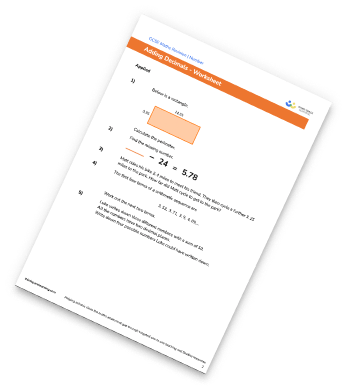Subtracting decimals worksheet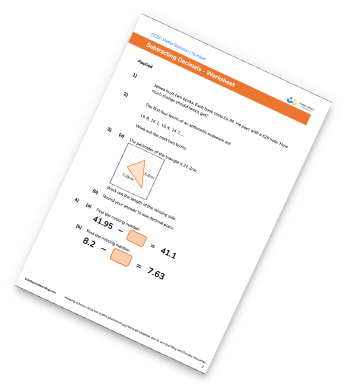Ordering fractions worksheet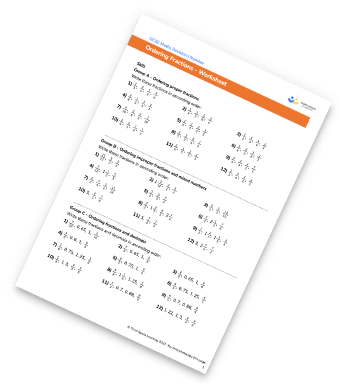Rational And Irrational Numbers Worksheet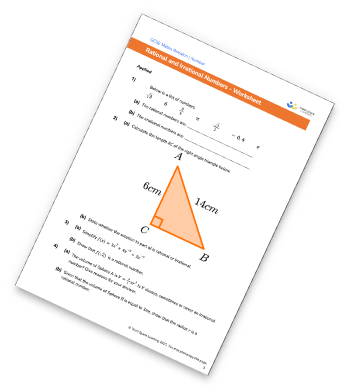## Do you have KS4 students who need more focused attention to succeed at GCSE?There will be students in your class who require individual attention to help them succeed in their maths GCSEs. In a class of 30, it’s not always easy to provide.

Help your students feel confident with exam-style questions and the strategies they’ll need to answer them correctly with our dedicated GCSE maths revision programme.

Lessons are selected to provide support where each student needs it most, and specially-trained GCSE maths tutors adapt the pitch and pace of each lesson. This ensures a personalised revision programme that raises grades and boosts confidence.

Find out more